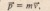# REVIEW AND SUMMARY

REVIEW  AND SUMMARY

Center of Mass The center of mass of a system of n particles is defined to be the point whose coordinates are given byLinear Momentum and Newton’s Second Law For a single particle, we define a quantity p called its linear momentum asConservation of Linear Momentum If a system is isolated so that no net external force acts on the system, the linear momentum P of the system remains constant.

Variable-Mass Systems If a system has varying mass, we redefine the system, enlarging its boundaries until they encompass a larger system whose mass does remain constant; then we apply the law of conservation of linear momentum.

External Forces and Internal Energy Changes Energy can transferred inside a system between an internal energy and mechanical energy via an external force F. The change AE.